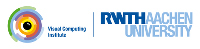OpenMesh
Using iterators and circulators

This examples shows:

• How to use iterators,
• How to use circulators.

This example is the first version of the simple mesh smoother. Here we will introduce iterators and circulators. These two concepts provide functionality to linearly enumerate e.g. all vertices of a mesh, and to circulate around a vertex, i.e. to enumerate all its one-ring neighbors. For a more detailed description, see Mesh Iterators and Circulators.

First we have to define the mesh type we want to use. This time we use a triangle mesh instead of a polygonal mesh:

#include <OpenMesh/Core/Mesh/TriMesh_ArrayKernelT.hh>
Triangle mesh based on the ArrayKernel.
Definition: TriMesh_ArrayKernelT.hh:96
Polygonal mesh based on the ArrayKernel.
Definition: PolyMesh_ArrayKernelT.hh:96

We read the mesh to be smoothed from a file:

if ( ! OpenMesh::IO::read_mesh(mesh, argv) )
bool read_mesh(Mesh &_mesh, const std::string &_filename)
Read a mesh from file _filename.
Definition: MeshIO.hh:95

One smoothing iteration is done in two steps:

1. For each vertex: calculate the barycenter of its one-ring neighbors.
2. For each vertex: move the vertex to the computed barycenter.

This can easily be implemented using vertex iterators. The mesh provides begin and end iterators by `vertices_begin()` and `vertices_end()`.

MyMesh::VertexIter v_it, v_end(mesh.vertices_end());
Kernel::VertexIter VertexIter
Scalar type.
Definition: PolyMeshT.hh:143
for (v_it=mesh.vertices_begin(); v_it!=v_end; ++v_it)

For calculating the barycenter, we have to iterate through the one-ring neighborhood of the current vertex. This functionality is provided by the `VertexVertexIter`:

Kernel::VertexVertexIter VertexVertexIter
Circulator.
Definition: PolyMeshT.hh:162
for (vv_it=mesh.vv_iter( *v_it ); vv_it.is_valid(); ++vv_it)

Now we can calculate the barycenters for each vertex and store them in the array `cogs`:

std::vector<MyMesh::Point> cogs;
for (v_it=mesh.vertices_begin(); v_it!=v_end; ++v_it)
{
cog = cog = cog = valence = 0.0;
for (vv_it=mesh.vv_iter( *v_it ); vv_it.is_valid(); ++vv_it)
{
cog += mesh.point( *vv_it );
++valence;
}
cogs.push_back(cog / valence);
}

After we have calculated the barycenters all that is left to do is to move the vertices to the corresponding barycenters. The complete source code is listed below.

#include <iostream>
#include <vector>
// -------------------- OpenMesh
#include <OpenMesh/Core/IO/MeshIO.hh>
#include <OpenMesh/Core/Mesh/TriMesh_ArrayKernelT.hh>
int main(int argc, char **argv)
{
MyMesh mesh;
// check command line options
if (argc != 4)
{
std::cerr << "Usage: " << argv << " #iterations infile outfile\n";
return 1;
}
if ( ! OpenMesh::IO::read_mesh(mesh, argv) )
{
std::cerr << "Error: Cannot read mesh from " << argv << std::endl;
return 1;
}
// this vector stores the computed centers of gravity
std::vector<MyMesh::Point> cogs;
std::vector<MyMesh::Point>::iterator cog_it;
cogs.reserve(mesh.n_vertices());
// smoothing mesh argv times
MyMesh::VertexIter v_it, v_end(mesh.vertices_end());
MyMesh::Scalar valence;
unsigned int i, N(atoi(argv));
for (i=0; i < N; ++i)
{
cogs.clear();
for (v_it=mesh.vertices_begin(); v_it!=v_end; ++v_it)
{
cog = cog = cog = valence = 0.0;
for (vv_it=mesh.vv_iter( *v_it ); vv_it.is_valid(); ++vv_it)
{
cog += mesh.point( *vv_it );
++valence;
}
cogs.push_back(cog / valence);
}
for (v_it=mesh.vertices_begin(), cog_it=cogs.begin();
v_it!=v_end; ++v_it, ++cog_it)
if ( !mesh.is_boundary( *v_it ) )
mesh.set_point( *v_it, *cog_it );
}
// write mesh to stdout
if ( ! OpenMesh::IO::write_mesh(mesh, argv) )
{
std::cerr << "Error: cannot write mesh to " << argv << std::endl;
return 1;
}
return 0;
}
bool write_mesh(const Mesh &_mesh, const std::string &_filename, Options _opt=Options::Default, std::streamsize _precision=6)
Write a mesh to the file _filename.
Definition: MeshIO.hh:190
Kernel::Scalar Scalar
Scalar type.
Definition: PolyMeshT.hh:110
Kernel::Point Point
Coordinate type.
Definition: PolyMeshT.hh:112

Project OpenMesh, ©  Visual Computing Institute, RWTH Aachen. Documentation generated using doxygen .